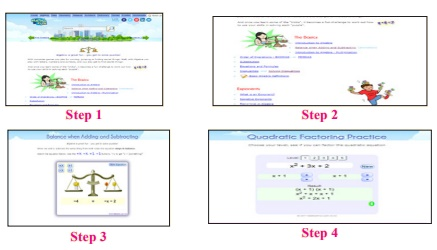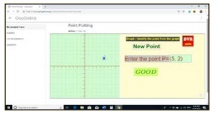Home | | Maths 8th Std | Summary

# Summary

Algebra | Chapter 3 | 8th Maths : Summary

SUMMARY

• When the product of two algebraic expressions we follow,

Multiply the signs of the terms,

Multiply the corresponding co-efficients of the terms.

Multiply the variable factors by using laws of exponents.

• For dividing a polynomial by a monomial, divide each term of the polynomial by a monomial.

• Identity: An identity is an equation is satisfied by any value that replaces its variables (s).

(a + b)2 = a 2 + 2ab + b2

( x + a)( x + b) = x 2 + (a + b)x + ab

(a - b)2 a 2 - 2ab b2

(a + b)3 = a 3 + 3a2b + 3ab 2 + b3

a 2 b2 = ( a + b)( a b)

(a b)3 = a 3 3a2b + 3ab 2 b3

( x + b )( x + b)( x + c) = x 3 + ( a + b + c)x 2 + ( ab + bc + ca )x + abc

• Factorisation: Expressing an algebraic expression as the product of two or more expression is called Factorisation.

• An equation containing only one variable with its highest power as one is called a linear equation in one variable.

• The value which replaces a variable in an equation so as to make the two sides of the equation equal is called a solution or root of the equation.

• Graphing is just a visual method for showing relationships between numbers.

• The horizontal line is named as XOX’, called the X-axis. The vertical line is named as YOY’, called the Y-axis. Both the axes are called coordinate axes. The plane containing the x axis and the y axis is known as the coordinate plane or the Cartesian plane.

• A point is denoted by a pair (a,b) of two numbers ‘a’ and ‘b’ listed in a specific order in which ‘a’ represents the distance along the X-axis and ‘b’ represents the distance along the Y axis. It is called an ordered pair (a,b).

• The coordinate axes divide the plane of the graph into four regions called quadrants.

• The line graph for the linear equation is called a linear graph.

ICT CORNER

Through this activity you will know about the Algebra, operations on them and study their properties as well.Step-1 Open the Browser and type the URL given below.

Step-2 Click on any one of the link in the items to know about the basics in algebra, exponents, polynomial, quadric equation etc.

Step-3 For example, click on the “Balance While adding and subtracting”, link under the Basic menu. A new tab will open in the browser where you can see the interactive game on adding and subtracting algebra.

Step-4 Likewise you can learn all the concepts in algebra.Web URL Algebra:

https://www.mathsisfun.com/algebra/index.html

*Pictures are indicatives only.

*If browser requires, allow Flash Player or Java Script to load the page.

ICT CORNER

Expected OutcomeStep-1 Open the Browser type the URL Link given below (or) Scan the QR Code. GeoGebra work book named “ALGEBRA” will open. Click on the worksheet named “Point Plotting”.

Step-2 In the given worksheet you can get new point by clicking on “New point”.Enter the correct point in the input box and press enter.